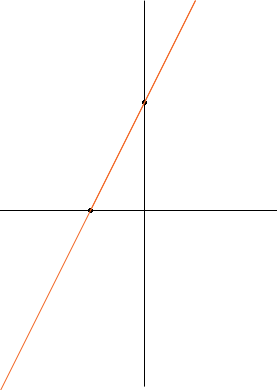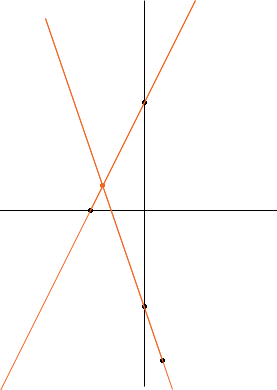SEARCH HOMEMath Central Quandaries & QueriesQuestion from Kate, a student: represent graphically the equations of these lines and their intersecting points x-y=2 3x+y=11Hi Kate,

I am going to illustrate with two different equations,

y - 2x = 6
3x + y = -5

Since you know these equations represent straight lines you only need two points on each line to sketch the graphs. For the first equation when x = 0, y = 6 so (0, 6) is a point on the graph. Likewise when y = 0, x = -3 so (-3, 0) is another point on the graph. Hence I can sketch the graph of y - 2x = 6For the second equation when x = 0, y = -5 so (0, -5) is a point on the graph. When y = 0 x is a fraction so I am going to choose a different point. When x = 1, y = -8 so (1, -8) is a point on the graph. Now when I plot both points on the same graph I can see the intersection point.Now try your equations. I have been sloppy and not put a scale on the axis. You need to make sure you include a scale.

PennyMath Central is supported by the University of Regina and The Pacific Institute for the Mathematical Sciences.